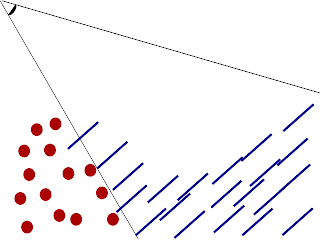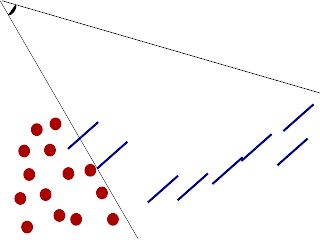## Saturday, April 2, 2011

### Screen Space Fluid rendering Phase 1

As I mentioned in my previous post, I have currently coded three of the necessary phases for rendering a set of points as fluid surface. The technique used is referred to as "Screen Space Fluid Rendering." The three phases are "Render points as Spheres", "Smooth the depth buffer", and finally "Calculate normals from the Smoothed Depth Buffer". In this blog, I will elaborate more on the screen space fluid rendering technique and I will discuss the first step.

Introduction to Screen Space Fluid Rendering(SSFR)

Before going into the details of each phase, I would like to introduce a little theory behind SSFR. The primary goal behind SSFR is to reconstruct the fluid surface in the viewer's (camera's) space. Concerning ourselves with only the viewer's perspective have potential speed up over methods like marching cubes which attempt to reconstruct the fluids entire surface. SSFR is not without limitations but for generating real time fluid animations it is among the fastest and highest quality currently available. An improvement in the smoothing phase, was developed and titled SSFR with Curvature flow.Figure 1: The viewer typically can only see a subset of the particles. Also, they can almost never see the opposite surface. These factors motivate the need for creating the surface in user's perspective only.Figure 2: Points outside the viewer's perspective are clipped. This is another way to save time.Figure 3: The green line represents the surface we hope to obtain by the end phase.
Creating Spheres from points

The first phase is to create spheres from a collection  of points. If we choose to actually render sphere meshes at each point the algorithm would become very expensive as the number of particles grows. Instead we choose to employ a GLSL shader similar to the one found in Nvidia's oclParticle demo. We only need to use a shader because after this phase we no longer use vertex information. All subsequent phases take output from previous phases and process the image.

uniform float pointRadius;
uniform float pointScale;   // scale to calculate size in pixels

varying vec3 posEye;        // position of center in eye space

void main()
{

posEye = vec3(gl_ModelViewMatrix * vec4(gl_Vertex.xyz, 1.0));
float dist = length(posEye);
gl_PointSize = pointRadius * (pointScale / dist);

gl_TexCoord = gl_MultiTexCoord0;
gl_Position = gl_ModelViewProjectionMatrix * vec4(gl_Vertex.xyz, 1.0);

gl_FrontColor = gl_Color;
}


uniform float pointRadius;  // point size in world space
uniform float near;
uniform float far;
varying vec3 posEye;        // position of center in eye space

void main()
{
// calculate normal from texture coordinates
vec3 n;
n.xy = gl_TexCoord.st*vec2(2.0, -2.0) + vec2(-1.0, 1.0);
//This is a more compatible version which works on ATI and Nvidia hardware
//However, This does not work on Apple computers. :/
//n.xy = gl_PointCoord.st*vec2(2.0, -2.0) + vec2(-1.0, 1.0);

float mag = dot(n.xy, n.xy);
if (mag > 1.0) discard;   // kill pixels outside circle
n.z = sqrt(1.0-mag);

// point on surface of sphere in eye space

vec4 clipSpacePos = gl_ProjectionMatrix*spherePosEye;
float normDepth = clipSpacePos.z/clipSpacePos.w;

// Transform into window coordinates coordinates
gl_FragDepth = (((far-near)/2.)*normDepth)+((far+near)/2.);
gl_FragData = gl_Color;
}


NOTE: The fragment shader above has some compatibility issues with ATI cards. Apparently the appropriate way to handle a point sprites texture coordinates is through gl_PointCoord. However this is not compatible with Apples OpenGL implementation.Figure 4: Turn the points from the Figure 2 into point sprites. Point sprites are useful because they always face the viewer.Figure 5: Point sprites which are "below" the surface do not need to be rendered.
The main goal of this shader is to modify the depth values of the rasterized image. To do this we must determine the z value from a 2D texture coordinate. First, take a look at the formula for a sphere.

$x^2+y^2+z^2 = 1$

Notice that we are outside the sphere if the following condition occurs:

$x^2 + y^2 > 0$

...
// calculate normal from texture coordinates
vec3 n;
n.xy = gl_TexCoord.st*vec2(2.0, -2.0) + vec2(-1.0, 1.0);
float mag = dot(n.xy, n.xy);
if (mag > 1.0) discard;   // kill pixels outside circle
...


If we are in the sphere then the following calculation will give us the z value of the point on the sphere.

$z = \sqrt{1-x^2-y^2}$

...
n.z = sqrt(1.0-mag);
...


The z value from this calculation is normalized on the interval [0,1]. The next step is to project this back into 3D camera coordinates. After projecting it into camera coordinates we must manually transform it back into window coordinates.

...
// point on surface of sphere in camera space

vec4 clipSpacePos = gl_ProjectionMatrix*spherePosEye;
float normDepth = clipSpacePos.z/clipSpacePos.w;

// Transform into window coordinates coordinates
gl_FragDepth = (((far-near)/2.)*normDepth)+((far+near)/2.);
...Figure 6: Morph the point sprites into hemispheres.

In my next post, I plan to explain how to modify the depth values in a way to make these bumpy spheres look more like a continuous surface.

All shader code and c++ code are available from enjalot's github repository. Rendering code can be found in rtps/rtpslib/Render/.